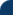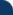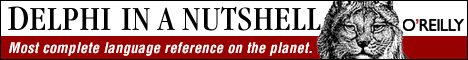Delphi Clinic C++Builder Gate Training & Consultancy Delphi Notes Weblog Dr.Bob's WebshopUnder The Hood #4 - Is the VCL thread-safe? See Also: Delphi Papers and Dr.Bob's Examines Columns

This time, we're taking a closer look at Delphi 2 and the inner workings of 32-bit Windows. Win32, a true pre-emptive operating system ads the new concept of multi-threading to our arsenal of programming techniques.
However, with each new technique, we must first master it before we can add it to our daily set of tools. So also with multi-threading. And one of the questions that comes up when associating threads with Delphi is the question: "Is the VCL thread-safe or not?"

The answer to this question seems not to be an easy one. Nor does it seem to be an absolute one. On one hand, Borland (and some books) can be heard to claim that the VCL is in fact thread safe. On the other hand are Delphi Experts and other books that claim the opposite. Why do people disagree on this already complex enough topic? To understand this, we have to take a deeper look under the hood of the TThread class in the VCL itself.

The TThread class lets you create multiple threads of execution in your Delphi application. Like Experts and other abstract classes in Delphi, TThread can't be used as-is. You must derive a new thread class from TThread (for example TDrBobThread) and override methods to use it. One of the methods is the Execute, and the other one is Synchronize.

Execute
Execute is the method where we must put the code for the thread to execute. Returning from Execute terminates the thread, frees the thread's stack, and calls the optional OnTerminate event handler (see the on-line for details). Our Execute method must periodically check the Terminated property; because if this is set to True, we must return immediately (otherwise our thread won't terminate correctly when the Terminate method is called).

Synchronize
The Synchronize procedure has one argument of type TThreadMethod. Synchronize lets you call this method of your thread object to avoid multi-thread conflicts with global VCL components. (VCL components can only be used from the main VCL thread; Synchronize calls the method you specify from within the main VCL thread so you can freely use properties and call methods of VCL components).

It is the Synchronized method that is allowed to use the global VCL visual components and call methods of these components. And if we add multiple threads to our application, we can safely access all VCL components, and call their methods as long as we only do so within the method we passed to Synchronize.

So, while we could say that the VCL as-is may not be thread-safe (i.e. you cannot just access VCL components in two threads' Execute method simultaneously), we have just seen a way to make sure we can use the VCL in a thread-safe way, by passing a custom method to the Synchronize procedure and use the global VCL components from there.

So, the VCL itself may not be thread-safe, but we can use it in a thread-safe way!

See the DEMOS/THREADS directory in your Delphi 2 installation for a nice multi-threaded sorting example (bubble sort vs. selection sort vs. quick sort), and notice how the VCL components are only used from within the Synchronized method DoVisualSwap.

For those of you who want to see the main SORTTHDS.PAS file, here it is:
```  unit SortThds;
interface
uses
Classes, Graphics, ExtCtrls;

Type

PSortArray = ^TSortArray;
TSortArray = array[0..MaxInt div SizeOf(Integer) - 1] of Integer;

private
FBox: TPaintBox;
FSortArray: PSortArray;
FSize: Integer;
FA, FB, FI, FJ: Integer;
procedure DoVisualSwap;
protected
procedure Execute; override;
procedure VisualSwap(A, B, I, J: Integer);
procedure Sort(var A: array of Integer); virtual; abstract;
public
constructor Create(Box: TPaintBox; var SortArray: array of Integer);
end;

{ TBubbleSort }

protected
procedure Sort(var A: array of Integer); override;
end;

{ TSelectionSort }

protected
procedure Sort(var A: array of Integer); override;
end;

{ TQuickSort }

protected
procedure Sort(var A: array of Integer); override;
end;

procedure PaintLine(Canvas: TCanvas; I, Len: Integer);

implementation

procedure PaintLine(Canvas: TCanvas; I, Len: Integer);
begin
Canvas.PolyLine([Point(0, I * 2 + 1), Point(Len, I * 2 + 1)]);
end;

constructor TSortThread.Create(Box: TPaintBox; var SortArray: array of Integer);
begin
inherited Create(False);
FBox := Box;
FSortArray := @SortArray;
FSize := High(SortArray) - Low(SortArray) + 1;
FreeOnTerminate := True;
end;

{ Since DoVisualSwap uses a VCL component (i.e., the TPaintBox) it should never
be called directly by this thread.  DoVisualSwap should be called by passing
it to the Synchronize method which causes DoVisualSwap to be executed by the
example of calling Synchronize. }

begin
with FBox do
begin
Canvas.Pen.Color := clBtnFace;
PaintLine(Canvas, FI, FA);
PaintLine(Canvas, FJ, FB);
Canvas.Pen.Color := clRed;
PaintLine(Canvas, FI, FB);
PaintLine(Canvas, FJ, FA);
end;
end;

{ VisusalSwap is a wrapper on DoVisualSwap making it easier to use.  The
parameters are copied to instance variables so they are accessable
by the main VCL thread when it executes DoVisualSwap }

procedure TSortThread.VisualSwap(A, B, I, J: Integer);
begin
FA := A;
FB := B;
FI := I;
FJ := J;
Synchronize(DoVisualSwap);
end;

{ The Execute method is called when the thread starts }

begin
Sort(Slice(FSortArray^, FSize));
end;

{ TBubbleSort }

procedure TBubbleSort.Sort(var A: array of Integer);
var
I, J, T: Integer;
begin
for I := High(A) downto Low(A) do
for J := Low(A) to High(A) - 1 do
if A[J] > A[J + 1] then
begin
VisualSwap(A[J], A[J + 1], J, J + 1);
T := A[J];
A[J] := A[J + 1];
A[J + 1] := T;
if Terminated then Exit;
end;
end;

{ TSelectionSort }

procedure TSelectionSort.Sort(var A: array of Integer);
var
I, J, T: Integer;
begin
for I := Low(A) to High(A) - 1 do
for J := High(A) downto I + 1 do
if A[I] > A[J] then
begin
VisualSwap(A[I], A[J], I, J);
T := A[I];
A[I] := A[J];
A[J] := T;
if Terminated then Exit;
end;
end;

{ TQuickSort }

procedure TQuickSort.Sort(var A: array of Integer);

procedure QuickSort(var A: array of Integer; iLo, iHi: Integer);
var
Lo, Hi, Mid, T: Integer;
begin
Lo := iLo;
Hi := iHi;
Mid := A[(Lo + Hi) div 2];
repeat
while A[Lo] < mid do inc(lo);
while a[hi] > Mid do Dec(Hi);
if Lo <= hi then
begin
VisualSwap(A[Lo], A[Hi], Lo, Hi);
T := A[Lo];
A[Lo] := A[Hi];
A[Hi] := T;
Inc(Lo);
Dec(Hi);
end;
until Lo > Hi;
if Hi > iLo then QuickSort(A, iLo, Hi);
if Lo < ihi then QuickSort(A, Lo, iHi);
if Terminated then Exit;
end;

begin
QuickSort(A, Low(A), High(A));
end;

end.
```